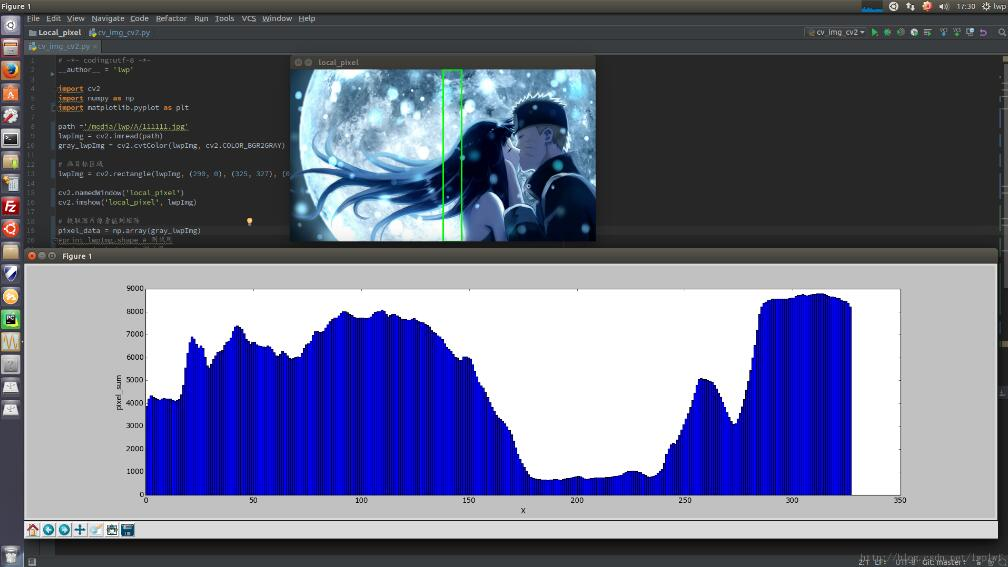## 您现在的位置是：门户> 编程语言> Python

Python+OpenCV图片局部区域像素值处理改进版详解
2020-08-14 114人围观 0条评论

上个版本的Python OpenCV图片局部区域像素值处理，虽然实现了我需要的功能，但还是走了很多弯路，我意识到图片本就是数组形式，对于8位灰度图，通道数为1,它就是个二位数组，这样就没有必要再设置ROI区域，复制出来这块区域再循环提取像素存入数组进行处理了，可以直接将图片存入数组，再利用numpy进行切分相应的数组操作就可以了，这样一想就简单很多了，这篇我会贴出修改后的代码，直接省去了大段的代码啊。

ps：这次我重新装的opencv3.2.0版本，代码里面直接用cv2了

```# 查看opencv版本，终端输入：
\$ pkg-config --modversion opencv```

cv_img_cv2.py

```# -*- coding:utf-8 -*-
__author__ = 'lwp'

import cv2
import numpy as np
import matplotlib.pyplot as plt

path ='/media/lwp/A/111111.jpg' # 图片路径
lwpImg = cv2.imread(path) # 加载图片
gray_lwpImg = cv2.cvtColor(lwpImg, cv2.COLOR_BGR2GRAY) # 转为灰度图

# 画目标区域，参数分别为图片、左上坐标、右下坐标、框的颜色、框线条的粗细
lwpImg = cv2.rectangle(lwpImg, (290, 0), (310, 327), (0, 255, 0), 2)
# 显示标记后的图片
cv2.imshow('local_pixel', lwpImg)

# 提取图片像素值到矩阵
pixel_data = np.array(gray_lwpImg)
# 提取目标区域
box_data = pixel_data[:, 290:310]
# 矩阵行求和
pixel_sum = np.sum(box_data, axis=1)

# 画图
x = range(576)
fig = plt.figure(figsize=(4, 2))
ax1 = fig.add_subplot(1, 1, 1)
ax1.bar(x, pixel_sum, width=1) # x为每个条形到x轴0点的距离，width为每个条的宽度
plt.xlabel('X')
plt.ylabel('Y')
plt.title('edge_filter')
plt.grid(True)
plt.show()

key = cv2.waitKey(0) & 0xFF
if key == ord('q'): # 按q关闭窗口
cv2.destroyAllWindows()```

效果：以上就是本文的全部内容，希望对大家的学习有所帮助，也希望大家多多支持脚本之家。

您可能感兴趣的文章:

## 文章评论

### 标签云